It has been an exciting few months developing Ready, our open-source program for exploring continuous cellular automata and reaction-diffusion systems. Version 0.5 has now been released, for Windows, MacOS and Linux. Go and try it out!

One change is that we now support normal (discrete) cellular automata in a more natural way, despite the fact that we're storing floats internally. Here's the OpenCL code for Frank Buss' hexagonal life-life rule:

```__kernel void rd_compute(
__global float* a_in,
__global float* a_out,
__global int* neighbor_indices,
__global float* neighbor_weights,
const int max_neighbors)
{
const int x = get_global_id(0);

// count neighbors of each type
int n1=0,n2=0;
int offset = x * max_neighbors;
for(int i=0;i<max_neighbors;i++)
{
if(neighbor_weights[offset+i]<0.0001f)
continue; // not a neighbor
int y = round(a_in[neighbor_indices[offset+i]]);
if(y==1) n1++;
else if(y==2) n2++;
}

// update step
int a = round(a_in[x]);
if(n1==0 && n2==1 && a==0)  a_out[x] = 2.0f;
else if(n1==1 && n2==1)     a_out[x] = 1.0f;
else if(n2==2)              a_out[x] = 1.0f;
else if(n2==3)              a_out[x] = 2.0f;
else                        a_out[x] = 0.0f;
}```

Given that this code will run on arbitrary meshes, with a variable number of neighbors, I think it's pretty readable!

Here are some of the cellular automata patterns we now include. Our support for arbitrary meshes means that we can run CA on Penrose tilings - the third image shows a new rule discovered in the last few months that supports gliders.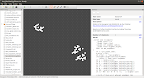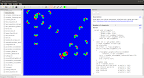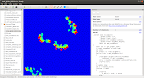Here's an animation of that Penrose rule, discovered by Katsunobu Imai:Another big change is that we now support polyhedral meshes. The most familiar of these are the cubes, but we also support three more kinds, as mentioned in a previous post. You can run cellular automata on them, as well as reaction-diffusion systems. This area is completely unexplored, so let us know what you find! Here's Gray-Scott running on a truncated octahedral honeycomb, contoured at 0.25: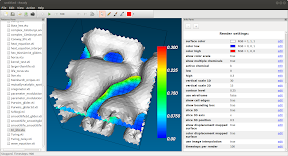Our use of OpenCL for the rules means that Ready can be programmed to do almost anything. Version 0.5 includes some SmoothLife rules, by Stephan Rafler. A video made by Ready of the SmoothLifeL rule went viral in October 2012, with 130,000 views on YouTube and discussions in several places.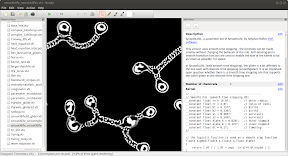Other rules supporting gliders are now included, such as this 3-chemical reaction-diffusion system discovered in 1999:

Robert Munafo's amazing U-Skate World continues to be an inspiration. Here are some videos showing various gliders, in 2D and 3D:

Here's the full changelist for Ready: changes.html